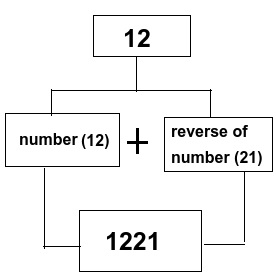# Find the Nth Even Length Palindrome using C++

If you ever used C + +, then you must have heard about Palindrome numbers. So in this guide, we will explain everything about "Nth even-length Palindrome" using appropriate examples. Palindrome numbers are numbers that stay the same after reversing them. Not only numbers but a word whose spelling stays the same when its characters are reversed. For Example −

Numbers = {1,121,131,656,1221,1551}

Words = {saas,malayalam,level,mom}

It looks complicated but very easy to perform on any system. So let's discuss the palindrome in brief.

## Nth Even length Palindrome Number

11,22,33,44,55,66,77,88,99,1001, etc. are some examples of even length palindrome numbers. We can also define it as first-half digits should be equal to second-half digits.

## How to find Nth Even Length Palindrome Number?

To find an even length palindrome number, we need to represent the number (given by the user) in two parts. We have to make sure that the first half should be equal to the second half, or we need to concatenate the number with its reversed value. To understand better, let's take an example

Input = 12

Output = 1221

Explanation − 12 is not a palindrome number, so to convert it into palindrome, 12 is concatenated with 21(reverse of 12). We can understand with the given diagramLet us see the same program in C++ −

#include <bits/stdc++.h>
using namespace std;
int main() {
int n;
cin >> n; // Taking input from the user.

cout << n; // printing given number
while(n) // This while loop will print the number in rever
{
cout << n % 10; // Example n = 10. In first iteration n % 10 = 0,
n = n/ 10; // in second iteration n = 1, now our n % 10 = 1 so output
will be 01.
}
}

Once you execute the above function in the system, you have to provide input for an output. So in this example, we put 3, 56, 10 and got the output 33, 5665, 1001.

Input : 3
Output : 33
Input : 56
Output : 5665
Input : 10
Output : 1001

## Explanation of code

Let us understand the code in parts

cin >> n; // Taking input from the user.

cout << n; // printing given number

Here we are taking input from the user and firstly print the number as it is because the first half of the output is the same as the input.

while(n) // This while loop will print the number in rever
{
cout << n % 10; // Example n = 10. In first iteration n % 10 = 0,
n = n/ 10; // in second iteration n = 1, now our n % 10 = 1 so output
will be 01.
}

We need to concatenate the first half with the reverse of the number. In this while loop, we are extracting the last value with the mod function and printing it, then removing that digit to move to the second last digit for printing. In this way, we are representing the given number as reversed.

## Conclusion

So in this article, we understand palindrome numbers and Nth even palindrome numbers. We have explained the complete information and approach of finding the Nth even length palindrome number program. The above was the simplest way to understand palindrome numbers. Therefore, we hope this helps you in understanding the problem more accurately.